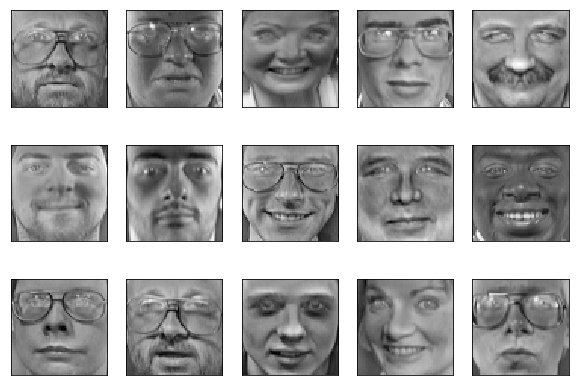In :
%matplotlib inline
import matplotlib.pyplot as plt

import numpy as np
from numpy import linalg

from sklearn.datasets import fetch_olivetti_faces


For this exercise, you will be making use of the Olivetti Faces dataset. Here is a description from the original website:

There are ten different images of each of 40 distinct subjects. For some subjects, the images were taken at different times, varying the lighting, facial expressions (open / closed eyes, smiling / not smiling) and facial details (glasses / no glasses). All the images were taken against a dark homogeneous background with the subjects in an upright, frontal position (with tolerance for some side movement).

In :
# load faces dataset
faces = fetch_olivetti_faces(shuffle=True).data

downloading Olivetti faces from https://ndownloader.figshare.com/files/5976027 to /Users/rasa/scikit_learn_data

In :
faces.shape  # 400 images of dimension 64 x 64
# note: images are elongated into a 4096-dimensional vector

Out:
(400, 4096)
In :
faces[np.random.randint(400)] #

Out:
array([0.42975205, 0.45041323, 0.49586776, ..., 0.30578512, 0.2892562 ,
0.3140496 ], dtype=float32)

For this exercise, we will be centering our dataset.

In :
faces = faces - faces.mean(axis=0)  # center data


In :
# Helper method: Plots the first 15 images in the dataset.
def plot_images(images,):
images = images[0:15,:]
plt.figure(figsize=(10,7))
for i, image in enumerate(images):
plt.subplot(3, 5, i+1)
cmap_range = max(image.max(), -image.min())
reshaped =  image.reshape((64,64))
plt.imshow(reshaped, vmin=-cmap_range, vmax=cmap_range,
cmap=plt.cm.gray, interpolation='nearest')
plt.xticks(())
plt.yticks(())


To get a sense of the dataset, let's first plot the some of the images:

In :
indexes = np.random.randint(0,400,15)
plot_images(faces)In [ ]:
"""
In this box, compute the SVD of the Faces dataset.
You may use the linalg module in numpy.
You should get three outputs, the left singular matrix U,
a vector of singular values S, and the transpose of the right
singular matrix, V_T.
"""
def compute_svd(data):
pass

U, S, V_T = compute_svd(faces)

In [ ]:
"""
In this box, print the dimensions of each of U, S, and V_T.
Are these dimensions in line with your expectations?
"""
pass

In [ ]:
"""
Now, print the first 15 elements of the vector of singular values.
How are they ordered?
"""
pass

In [ ]:
"""
Plot the first 15 right singular vectors of the Faces dataset.
What do you observe?
"""
pass


How are these singular vectors related to the dataset? Think about the relationship between SVD and eigendecomposition.